Documentation

# pwelch

Welch’s power spectral density estimate

## Syntax

pxx = pwelch(x)
pxx = pwelch(x,window)
pxx = pwelch(x,window,noverlap) 
pxx = pwelch(x,window,noverlap,nfft)
[pxx,w] = pwelch(___)
[pxx,f] = pwelch(___,fs)
[pxx,w] = pwelch(x,window,noverlap,w)
[pxx,f] = pwelch(x,window,noverlap,f,fs)
[___] = pwelch(x,window,___,freqrange)
[___] = pwelch(x,window,___,trace)
[___,pxxc] = pwelch(___,'ConfidenceLevel',probability)
[___] = pwelch(___,spectrumtype)
pwelch(___)

## Description

example

pxx = pwelch(x) returns the power spectral density (PSD) estimate, pxx, of the input signal, x, found using Welch's overlapped segment averaging estimator. When x is a vector, it is treated as a single channel. When x is a matrix, the PSD is computed independently for each column and stored in the corresponding column of pxx. If x is real-valued, pxx is a one-sided PSD estimate. If x is complex-valued, pxx is a two-sided PSD estimate. By default, x is divided into the longest possible segments to obtain as close to but not exceed 8 segments with 50% overlap. Each segment is windowed with a Hamming window. The modified periodograms are averaged to obtain the PSD estimate. If you cannot divide the length of x exactly into an integer number of segments with 50% overlap, x is truncated accordingly.

example

pxx = pwelch(x,window) uses the input vector or integer, window, to divide the signal into segments. If window is a vector, pwelch divides the signal into segments equal in length to the length of window. The modified periodograms are computed using the signal segments multiplied by the vector, window. If window is an integer, the signal is divided into segments of length window. The modified periodograms are computed using a Hamming window of length window.

example

pxx = pwelch(x,window,noverlap)  uses noverlap samples of overlap from segment to segment. noverlap must be a positive integer smaller than window if window is an integer. noverlap must be a positive integer less than the length of window if window is a vector. If you do not specify noverlap, or specify noverlap as empty, the default number of overlapped samples is 50% of the window length.

example

pxx = pwelch(x,window,noverlap,nfft) specifies the number of discrete Fourier transform (DFT) points to use in the PSD estimate. The default nfft is the greater of 256 or the next power of 2 greater than the length of the segments.
[pxx,w] = pwelch(___) returns the normalized frequency vector, w. If pxx is a one-sided PSD estimate, w spans the interval [0,π] if nfft is even and [0,π) if nfft is odd. If pxx is a two-sided PSD estimate, w spans the interval [0,2π).

example

[pxx,f] = pwelch(___,fs) returns a frequency vector, f, in cycles per unit time. The sample rate, fs, is the number of samples per unit time. If the unit of time is seconds, then f is in cycles/sec (Hz). For real–valued signals, f spans the interval [0,fs/2] when nfft is even and [0,fs/2) when nfft is odd. For complex-valued signals, f spans the interval [0,fs). fs must be the fifth input to pwelch. To input a sample rate and still use the default values of the preceding optional arguments, specify these arguments as empty, [].
[pxx,w] = pwelch(x,window,noverlap,w) returns the two-sided Welch PSD estimates at the normalized frequencies specified in the vector, w. The vector w must contain at least two elements, because otherwise the function interprets it as nfft.
[pxx,f] = pwelch(x,window,noverlap,f,fs) returns the two-sided Welch PSD estimates at the frequencies specified in the vector, f. The vector f must contain at least two elements, because otherwise the function interprets it as nfft. The frequencies in f are in cycles per unit time. The sample rate, fs, is the number of samples per unit time. If the unit of time is seconds, then f is in cycles/sec (Hz).

example

[___] = pwelch(x,window,___,freqrange) returns the Welch PSD estimate over the frequency range specified by freqrange. Valid options for freqrange are: 'onesided', 'twosided', or 'centered'.

example

[___] = pwelch(x,window,___,trace) returns the maximum-hold spectrum estimate if trace is specified as 'maxhold' and returns the minimum-hold spectrum estimate if trace is specified as 'minhold'. 

example

[___,pxxc] = pwelch(___,'ConfidenceLevel',probability) returns the probability × 100% confidence intervals for the PSD estimate in pxxc. 

example

[___] = pwelch(___,spectrumtype) returns the PSD estimate if spectrumtype is specified as 'psd' and returns the power spectrum if spectrumtype is specified as 'power'.

example

pwelch(___) with no output arguments plots the Welch PSD estimate in the current figure window. 

## Examples

collapse all

Obtain the Welch PSD estimate of an input signal consisting of a discrete-time sinusoid with an angular frequency of $\pi /4$ rad/sample with additive $N\left(0,1\right)$ white noise.

Create a sine wave with an angular frequency of $\pi /4$ rad/sample with additive $N\left(0,1\right)$ white noise. Reset the random number generator for reproducible results. The signal has a length ${N}_{x}=320$ samples.

rng default n = 0:319; x = cos(pi/4*n)+randn(size(n));

Obtain the Welch PSD estimate using the default Hamming window and DFT length. The default segment length is 71 samples and the DFT length is the 256 points yielding a frequency resolution of $2\pi /256$ rad/sample. Because the signal is real-valued, the periodogram is one-sided and there are 256/2+1 points. Plot the Welch PSD estimate.

pxx = pwelch(x); pwelch(x)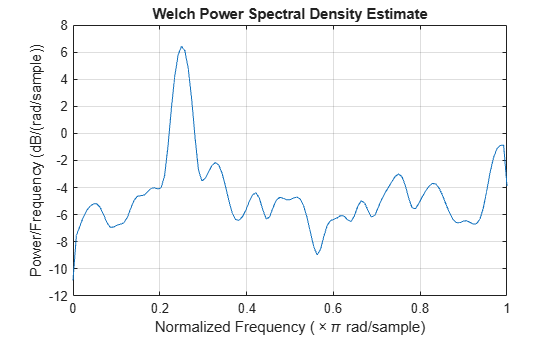Repeat the computation.

• Divide the signal into sections of length $nsc=⌊{N}_{x}/4.5⌋$. This action is equivalent to dividing the signal into the longest possible segments to obtain as close to but not exceed 8 segments with 50% overlap.

• Window the sections using a Hamming window.

• Specify 50% overlap between contiguous sections

• To compute the FFT, use $\mathrm{max}\left(256,{2}^{p}\right)$ points, where $p=⌈{\mathrm{log}}_{2}nsc⌉$.

Verify that the two approaches give identical results.

Nx = length(x); nsc = floor(Nx/4.5); nov = floor(nsc/2); nff = max(256,2^nextpow2(nsc)); t = pwelch(x,hamming(nsc),nov,nff); maxerr = max(abs(abs(t(:))-abs(pxx(:))))
maxerr = 0 

Divide the signal into 8 sections of equal length, with 50% overlap between sections. Specify the same FFT length as in the preceding step. Compute the Welch PSD estimate and verify that it gives the same result as the previous two procedures.

ns = 8; ov = 0.5; lsc = floor(Nx/(ns-(ns-1)*ov)); t = pwelch(x,lsc,floor(ov*lsc),nff); maxerr = max(abs(abs(t(:))-abs(pxx(:))))
maxerr = 0 

Obtain the Welch PSD estimate of an input signal consisting of a discrete-time sinusoid with an angular frequency of $\pi /3$ rad/sample with additive $N\left(0,1\right)$ white noise.

Create a sine wave with an angular frequency of $\pi /3$ rad/sample with additive $N\left(0,1\right)$ white noise. Reset the random number generator for reproducible results. The signal has 512 samples.

rng default n = 0:511; x = cos(pi/3*n)+randn(size(n));

Obtain the Welch PSD estimate dividing the signal into segments 132 samples in length. The signal segments are multiplied by a Hamming window 132 samples in length. The number of overlapped samples is not specified, so it is set to 132/2 = 66. The DFT length is 256 points, yielding a frequency resolution of $2\pi /256$ rad/sample. Because the signal is real-valued, the PSD estimate is one-sided and there are 256/2+1 = 129 points. Plot the PSD as a function of normalized frequency.

segmentLength = 132; [pxx,w] = pwelch(x,segmentLength); plot(w/pi,10*log10(pxx)) xlabel('\omega / \pi')Obtain the Welch PSD estimate of an input signal consisting of a discrete-time sinusoid with an angular frequency of $\pi /4$ rad/sample with additive $N\left(0,1\right)$ white noise.

Create a sine wave with an angular frequency of $\pi /4$ rad/sample with additive $N\left(0,1\right)$ white noise. Reset the random number generator for reproducible results. The signal is 320 samples in length.

rng default n = 0:319; x = cos(pi/4*n)+randn(size(n));

Obtain the Welch PSD estimate dividing the signal into segments 100 samples in length. The signal segments are multiplied by a Hamming window 100 samples in length. The number of overlapped samples is 25. The DFT length is 256 points yielding a frequency resolution of $2\pi /256$ rad/sample. Because the signal is real-valued, the PSD estimate is one-sided and there are 256/2+1 points.

segmentLength = 100; noverlap = 25; pxx = pwelch(x,segmentLength,noverlap); plot(10*log10(pxx))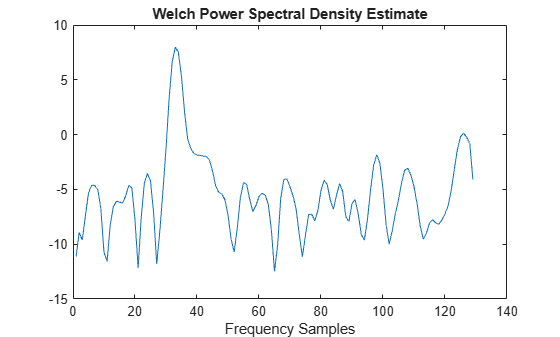Obtain the Welch PSD estimate of an input signal consisting of a discrete-time sinusoid with an angular frequency of $\pi /4$ rad/sample with additive $N\left(0,1\right)$ white noise.

Create a sine wave with an angular frequency of $\pi /4$ rad/sample with additive $N\left(0,1\right)$ white noise. Reset the random number generator for reproducible results. The signal is 320 samples in length.

rng default n = 0:319; x = cos(pi/4*n) + randn(size(n));

Obtain the Welch PSD estimate dividing the signal into segments 100 samples in length. Use the default overlap of 50%. Specify the DFT length to be 640 points so that the frequency of $\pi /4$ rad/sample corresponds to a DFT bin (bin 81). Because the signal is real-valued, the PSD estimate is one-sided and there are 640/2+1 points.

segmentLength = 100; nfft = 640; pxx = pwelch(x,segmentLength,[],nfft); plot(10*log10(pxx)) xlabel('rad/sample') ylabel('dB / (rad/sample)')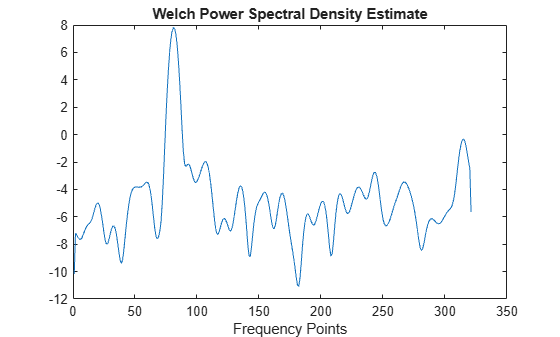Create a signal consisting of a 100 Hz sinusoid in additive N(0,1) white noise. Reset the random number generator for reproducible results. The sample rate is 1 kHz and the signal is 5 seconds in duration.

rng default fs = 1000; t = 0:1/fs:5-1/fs; x = cos(2*pi*100*t) + randn(size(t));

Obtain Welch's overlapped segment averaging PSD estimate of the preceding signal. Use a segment length of 500 samples with 300 overlapped samples. Use 500 DFT points so that 100 Hz falls directly on a DFT bin. Input the sample rate to output a vector of frequencies in Hz. Plot the result.

[pxx,f] = pwelch(x,500,300,500,fs); plot(f,10*log10(pxx)) xlabel('Frequency (Hz)') ylabel('PSD (dB/Hz)')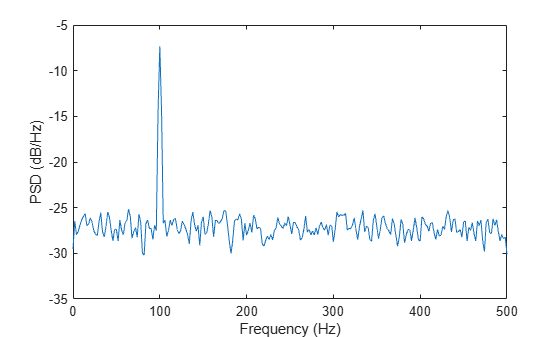Create a signal consisting of three noisy sinusoids and a chirp, sampled at 200 kHz for 0.1 second. The frequencies of the sinusoids are 1 kHz, 10 kHz, and 20 kHz. The sinusoids have different amplitudes and noise levels. The noiseless chirp has a frequency that starts at 20 kHz and increases linearly to 30 kHz during the sampling.

Fs = 200e3; Fc = [1 10 20]'*1e3; Ns = 0.1*Fs; t = (0:Ns-1)/Fs; x = [1 1/10 10]*sin(2*pi*Fc*t)+[1/200 1/2000 1/20]*randn(3,Ns); x = x+chirp(t,20e3,t(end),30e3);

Compute the Welch PSD estimate and the maximum-hold and minimum-hold spectra of the signal. Plot the results.

[pxx,f] = pwelch(x,[],[],[],Fs); pmax = pwelch(x,[],[],[],Fs,'maxhold'); pmin = pwelch(x,[],[],[],Fs,'minhold'); plot(f,pow2db(pxx)) hold on plot(f,pow2db([pmax pmin]),':') hold off xlabel('Frequency (Hz)') ylabel('PSD (dB/Hz)') legend('pwelch','maxhold','minhold')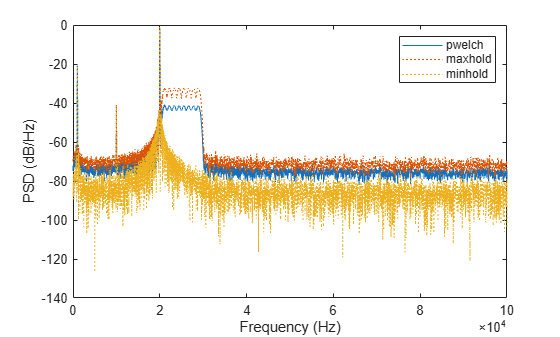Repeat the procedure, this time computing centered power spectrum estimates.

[pxx,f] = pwelch(x,[],[],[],Fs,'centered','power'); pmax = pwelch(x,[],[],[],Fs,'maxhold','centered','power'); pmin = pwelch(x,[],[],[],Fs,'minhold','centered','power'); plot(f,pow2db(pxx)) hold on plot(f,pow2db([pmax pmin]),':') hold off xlabel('Frequency (Hz)') ylabel('Power (dB)') legend('pwelch','maxhold','minhold')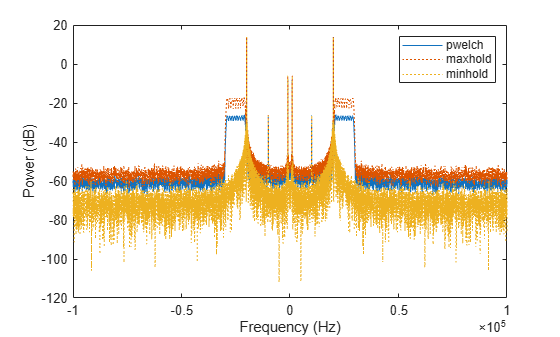This example illustrates the use of confidence bounds with Welch's overlapped segment averaging (WOSA) PSD estimate. While not a necessary condition for statistical significance, frequencies in Welch's estimate where the lower confidence bound exceeds the upper confidence bound for surrounding PSD estimates clearly indicate significant oscillations in the time series.

Create a signal consisting of the superposition of 100 Hz and 150 Hz sine waves in additive white N(0,1) noise. The amplitude of the two sine waves is 1. The sample rate is 1 kHz. Reset the random number generator for reproducible results.

rng default fs = 1000; t = 0:1/fs:1-1/fs; x = cos(2*pi*100*t)+sin(2*pi*150*t)+randn(size(t));

Obtain the WOSA estimate with 95%-confidence bounds. Set the segment length equal to 200 and overlap the segments by 50% (100 samples). Plot the WOSA PSD estimate along with the confidence interval and zoom in on the frequency region of interest near 100 and 150 Hz.

L = 200; noverlap = 100; [pxx,f,pxxc] = pwelch(x,hamming(L),noverlap,200,fs,... 'ConfidenceLevel',0.95); plot(f,10*log10(pxx)) hold on plot(f,10*log10(pxxc),'-.') hold off xlim([25 250]) xlabel('Frequency (Hz)') ylabel('PSD (dB/Hz)') title('Welch Estimate with 95%-Confidence Bounds')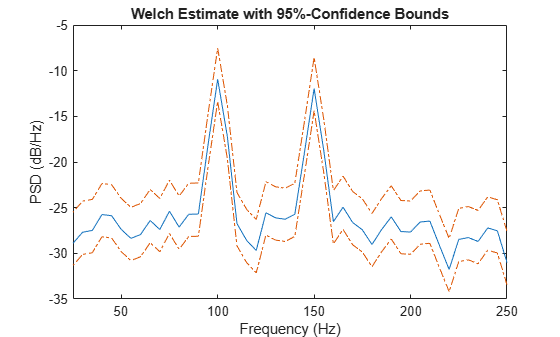The lower confidence bound in the immediate vicinity of 100 and 150 Hz is significantly above the upper confidence bound outside the vicinity of 100 and 150 Hz.

Create a signal consisting of a 100 Hz sinusoid in additive $N\left(0,1/4\right)$ white noise. Reset the random number generator for reproducible results. The sample rate is 1 kHz and the signal is 5 seconds in duration.

rng default fs = 1000; t = 0:1/fs:5-1/fs; noisevar = 1/4; x = cos(2*pi*100*t)+sqrt(noisevar)*randn(size(t));

Obtain the DC-centered power spectrum using Welch's method. Use a segment length of 500 samples with 300 overlapped samples and a DFT length of 500 points. Plot the result.

[pxx,f] = pwelch(x,500,300,500,fs,'centered','power'); plot(f,10*log10(pxx)) xlabel('Frequency (Hz)') ylabel('Magnitude (dB)') grid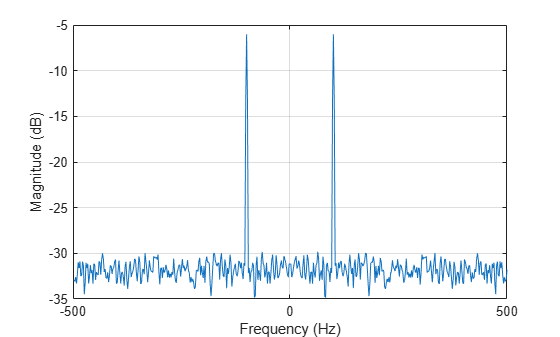You see that the power at -100 and 100 Hz is close to the expected power of 1/4 for a real-valued sine wave with an amplitude of 1. The deviation from 1/4 is due to the effect of the additive noise.

Generate 1024 samples of a multichannel signal consisting of three sinusoids in additive $N\left(0,1\right)$ white Gaussian noise. The sinusoids' frequencies are $\pi /2$, $\pi /3$, and $\pi /4$ rad/sample. Estimate the PSD of the signal using Welch's method and plot it.

N = 1024; n = 0:N-1; w = pi./[2;3;4]; x = cos(w*n)' + randn(length(n),3); pwelch(x)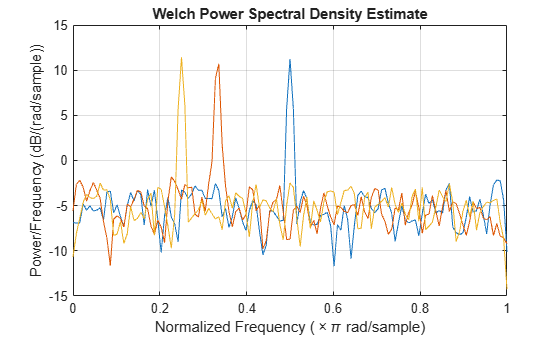## Input Arguments

collapse all

Input signal, specified as a row or column vector, or as a matrix. If x is a matrix, then its columns are treated as independent channels.

Example: cos(pi/4*(0:159))+randn(1,160) is a single-channel row-vector signal.

Example: cos(pi./[4;2]*(0:159))'+randn(160,2) is a two-channel signal.

Data Types: single | double
Complex Number Support: Yes

Window, specified as a row or column vector or an integer. If window is a vector, pwelch divides x into overlapping segments of length equal to the length of window, and then multiplies each signal segment with the vector specified in window. If window is an integer, pwelch is divided into segments of length equal to the integer value, and a Hamming window of equal length is used. If the length of x cannot be divided exactly into an integer number of segments with noverlap number of overlapping samples, x is truncated accordingly. If you specify window as empty, the default Hamming window is used to obtain eight segments of x with noverlap overlapping samples.

Data Types: single | double

Number of overlapped samples, specified as a positive integer smaller than the length of window. If you omit noverlap or specify noverlap as empty, a value is used to obtain 50% overlap between segments.

Number of DFT points, specified as a positive integer. For a real-valued input signal, x, the PSD estimate, pxx has length (nfft/2 + 1) if nfft is even, and (nfft + 1)/2 if nfft is odd. For a complex-valued input signal,x, the PSD estimate always has length nfft. If nfft is specified as empty, the default nfft is used.

If nfft is greater than the segment length, the data is zero-padded. If nfft is less than the segment length, the segment is wrapped using datawrap to make the length equal to nfft.

Data Types: single | double

Sample rate, specified as a positive scalar. The sample rate is the number of samples per unit time. If the unit of time is seconds, then the sample rate has units of Hz.

Normalized frequencies, specified as a row or column vector with at least two elements. Normalized frequencies are in rad/sample.

Example: w = [pi/4 pi/2]

Data Types: double

Frequencies, specified as a row or column vector with at least two elements. The frequencies are in cycles per unit time. The unit time is specified by the sample rate, fs. If fs has units of samples/second, then f has units of Hz.

Example: fs = 1000; f = [100 200]

Data Types: double

Frequency range for the PSD estimate, specified as a one of 'onesided', 'twosided', or 'centered'. The default is 'onesided' for real-valued signals and 'twosided' for complex-valued signals. The frequency ranges corresponding to each option are

• 'onesided' — returns the one-sided PSD estimate of a real-valued input signal, x. If nfft is even, pxx has length nfft/2 + 1 and is computed over the interval [0,π] rad/sample. If nfft is odd, the length of pxx is (nfft + 1)/2 and the interval is [0,π) rad/sample. When fs is optionally specified, the corresponding intervals are [0,fs/2] cycles/unit time and [0,fs/2) cycles/unit time for even and odd length nfft respectively.

• 'twosided' — returns the two-sided PSD estimate for either the real-valued or complex-valued input, x. In this case, pxx has length nfft and is computed over the interval [0,2π) rad/sample. When fs is optionally specified, the interval is [0,fs) cycles/unit time.

• 'centered' — returns the centered two-sided PSD estimate for either the real-valued or complex-valued input, x. In this case, pxx has length nfft and is computed over the interval (–π,π] rad/sample for even length nfft and (–π,π) rad/sample for odd length nfft. When fs is optionally specified, the corresponding intervals are (–fs/2, fs/2] cycles/unit time and (–fs/2, fs/2) cycles/unit time for even and odd length nfft respectively.

Power spectrum scaling, specified as one of 'psd' or 'power'. Omitting the spectrumtype, or specifying 'psd', returns the power spectral density. Specifying 'power' scales each estimate of the PSD by the equivalent noise bandwidth of the window. Use the 'power' option to obtain an estimate of the power at each frequency.

Trace mode, specified as one of 'mean', 'maxhold', or 'minhold'. The default is 'mean'.

• 'mean' — returns the Welch spectrum estimate of each input channel. pwelch computes the Welch spectrum estimate at each frequency bin by averaging the power spectrum estimates of all the segments.

• 'maxhold' — returns the maximum-hold spectrum of each input channel. pwelch computes the maximum-hold spectrum at each frequency bin by keeping the maximum value among the power spectrum estimates of all the segments.

• 'minhold' — returns the minimum-hold spectrum of each input channel. pwelch computes the minimum-hold spectrum at each frequency bin by keeping the minimum value among the power spectrum estimates of all the segments.

Coverage probability for the true PSD, specified as a scalar in the range (0,1). The output, pxxc, contains the lower and upper bounds of the probability × 100% interval estimate for the true PSD.

## Output Arguments

collapse all

PSD estimate, returned as a real-valued, nonnegative column vector or matrix. Each column of pxx is the PSD estimate of the corresponding column of x. The units of the PSD estimate are in squared magnitude units of the time series data per unit frequency. For example, if the input data is in volts, the PSD estimate is in units of squared volts per unit frequency. For a time series in volts, if you assume a resistance of 1 Ω and specify the sample rate in hertz, the PSD estimate is in watts per hertz.

Data Types: single | double

Normalized frequencies, returned as a real-valued column vector. If pxx is a one-sided PSD estimate, w spans the interval [0,π] if nfft is even and [0,π) if nfft is odd. If pxx is a two-sided PSD estimate, w spans the interval [0,2π). For a DC-centered PSD estimate, w spans the interval (–π,π] for even nfft and (–π,π) for odd nfft.

Data Types: double

Cyclical frequencies, returned as a real-valued column vector. For a one-sided PSD estimate, f spans the interval [0,fs/2] when nfft is even and [0,fs/2) when nfft is odd. For a two-sided PSD estimate, f spans the interval [0,fs). For a DC-centered PSD estimate, f spans the interval (–fs/2, fs/2] cycles/unit time for even length nfft and (–fs/2, fs/2) cycles/unit time for odd length nfft.

Data Types: double | single

Confidence bounds, returned as a matrix with real-valued elements. The row size of the matrix is equal to the length of the PSD estimate, pxx. pxxc has twice as many columns as pxx. Odd-numbered columns contain the lower bounds of the confidence intervals, and even-numbered columns contain the upper bounds. Thus, pxxc(m,2*n-1) is the lower confidence bound and pxxc(m,2*n) is the upper confidence bound corresponding to the estimate pxx(m,n). The coverage probability of the confidence intervals is determined by the value of the probability input.

Data Types: single | double

collapse all

### Welch’s Overlapped Segment Averaging Spectral Estimation

The periodogram is not a consistent estimator of the true power spectral density of a wide-sense stationary process. Welch’s technique to reduce the variance of the periodogram breaks the time series into segments, usually overlapping.

Welch’s method computes a modified periodogram for each segment and then averages these estimates to produce the estimate of the power spectral density. Because the process is wide-sense stationary and Welch’s method uses PSD estimates of different segments of the time series, the modified periodograms represent approximately uncorrelated estimates of the true PSD and averaging reduces the variability.

The segments are typically multiplied by a window function, such as a Hamming window, so that Welch’s method amounts to averaging modified periodograms. Because the segments usually overlap, data values at the beginning and end of the segment tapered by the window in one segment, occur away from the ends of adjacent segments. This guards against the loss of information caused by windowing.